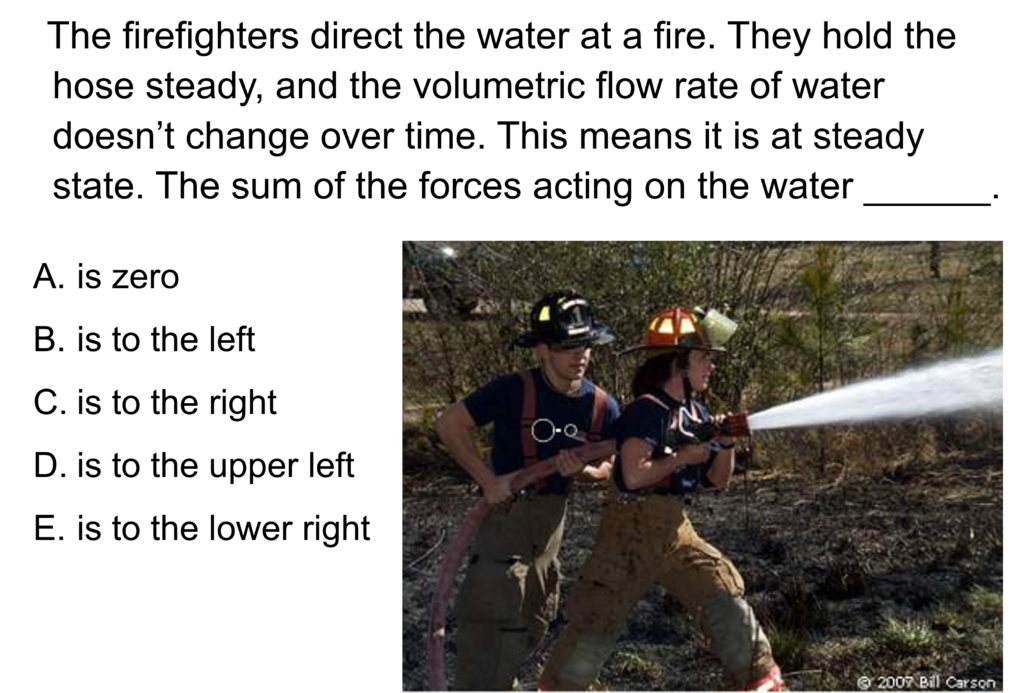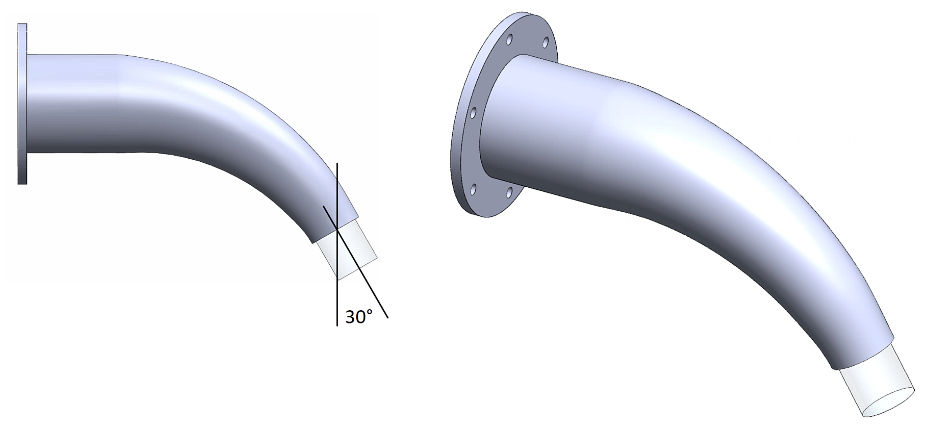LearnChemE

#### Linear Momentum: ConcepTest and Example Problem

Try to answer this ConcepTest and solve the example problem before using this module. Studies show that trying to answer the questions before studying material improves learning and retention. We suggest that you write down the reasons for your answers. By the end of this module, you should be able to answer these on your own. Answers will be given at the end of this module.##### Example Problem 1

The aluminum elbow is bolted to a pipe, which is not shown. The bolts provide a force that anchors the elbow in place. What are the x- and y-components of this anchoring force? The diameters of the entrance and exit are D1 = 10 cm and D2 = 5 cm. The mass of the elbow is me = 2.4 kg. The mass of the water inside of it is mw = 3 kg. The volumetric flow rate of the water is Q = 15 L/s. The gage pressure of the water entering the elbow is P1 = 30 kPa. The water exits the elbow as a free jet.